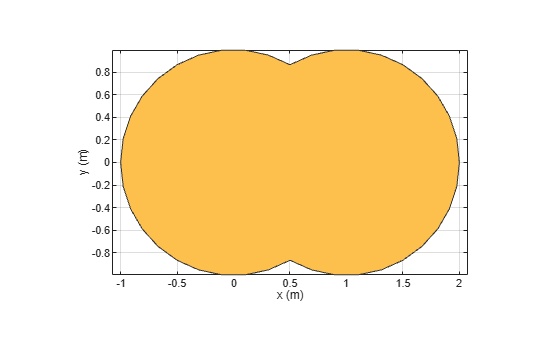Boolean unite operation on two shapes

Description

example

c = add(shape1,shape2) unites shape1 and shape2 using the add operation. You can also use the + to add the two shapes together.

Examples

collapse all

Create and view a default circle.

circle1 = antenna.Circle;

Create a circle with a radius of 1 m. The center of the circle is at [1 0].Create circle with a radius of 1 m. The center of the circle is at [1 0].

Create a rectangle with a length of 2 m and a width of 4 m centered at the origin.

rect1 = antenna.Rectangle('Length',2,'Width',2);

Add the two shapes together using the + function.

polygon1 = circle1+rect1
polygon1 =
Polygon with properties:

Name: 'mypolygon'
Vertices: [21x3 double]

show(polygon1)Input Arguments

collapse all

Shapes created using custom elements and shape objects of Antenna Toolbox™, specified as an object.

Example: c = add(rectangle1, rectangle2), where rectangle1 and rectangle2 are shapes created using antenna.Rectangle object.